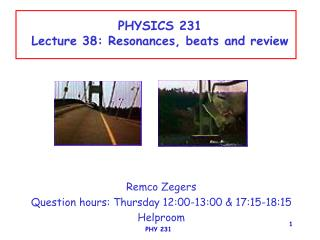DownloadDownload PresentationPHYSICS 231 Lecture 38: Resonances, beats and review

# PHYSICS 231 Lecture 38: Resonances, beats and review

Télécharger la présentation## PHYSICS 231 Lecture 38: Resonances, beats and review

- - - - - - - - - - - - - - - - - - - - - - - - - - - E N D - - - - - - - - - - - - - - - - - - - - - - - - - - -
##### Presentation Transcript

1. PHYSICS 231Lecture 38: Resonances, beats and review Remco Zegers Question hours: Thursday 12:00-13:00 & 17:15-18:15 Helproom PHY 231

2. standing waves in a rope both ends fixed n=2L/n or L=nn/2 F: tension in rope : mass per unit length f1: fundamental frequency PHY 231

3. Both ends open PHY 231

4. One end open, one end closed even harmonics are missing!!! PHY 231

5. f3=3*343/(4*0.5)=514 Hz f1=343/(4*0.5)=172 Hz f3=3*343/(4*0.25)=1028 Hz f1=343/(4*0.25)=343 Hz example A simple flute is played by blowing air in on one side and the other end is open. The length of the tube can be varied manually (like a trombone). What are the frequencies of the first two possible harmonics if L=0.5m? If the length is made half of the original length, how will these change v=343m/s? PHY 231

6. f2=2*343/(2*0.5)=686 Hz f1=343/(2*0.5)=343 Hz f2=2*343/(2*0.25)=1372 Hz f1=343/(2*0.25)=686Hz example A simple flute is played by blowing air in on one side and the other end is closed. The length of the tube can be varied manually (like a trombone). What are the frequencies of the first two possible harmonics if L=0.5m? If the length is made half of the original length, how will these change v=343m/s? PHY 231

7. harmonics Generally speaking, many harmonics with different intensities can be present at the same time. + L PHY 231

8. beats Superposition of 2 waves with slightly different frequency DEMO The amplitude changes as a function of time, so the intensity of sound changes as a function of time. The beat frequency (number of intensity maxima/minima per second): fbeat=|fa-fb| PHY 231

9. so f~F b) fcurrent/fideal= (Fcurrent/Fideal) 497/500=0.954 or 503/500=1.006 Fideal=Fcurrent/(0.994)2=1.012Fcurrent or Fideal=Fcurrent/(1.006)2=0.988Fcurrent example Someone is trying to tune a guitar. One of the strings is supposed to have a frequency of 500 Hz. The person is using a tuning fork which produces a sound of exactly this frequency, but while sounding the fork and the playing the guitar, hears a beat in the sound with a frequency of 3 Hz (3 beat per second). a) What is the real frequency of the guitar string? b) By what fraction does the person need to change the tension of the guitar string to tune it properly? a) fb=|ffork-fguitar| 3=|500-fguitar| fguitar=497 or 503 Hz PHY 231

10. Resonances Realistically, oscillations are damped due to frictional forces. However, we can drive the oscillation via an external source. Example: mass on a spring: natural frequency f=1/(2)(k/m) If the frequency of the driving force equals the natural frequency: large oscillations occur: Resonance demo • Resonances occur in many daily situations: • shock absorber in car • playing basketball • resonating lecture room!! Famous example: Tacoma bridge PHY 231

11. Review Start with the sample problems on the web to see how you stand on each chapter!! see also recitations on Thursday (12-1 and 17:15-18:15) review on Friday any more??? today: one problem each from ch. 2,3,4,5,6 Friday: one problem each from 7,8,9 and the rest 10,11,12,13,14 (2 each) PHY 231

12. x(t)=x(0)+v(0)t+½at2 v(t)=v(0)+at chapter 2. A person throws 2 stones from the top of a building with a speed of 20 m/s. One is thrown up, and the other is thrown down. The first one hits the street after 5 s. How much later does the second one hit? Stone thrown down: x(t)=x(0)+v(0)t+½at2=h-20t-½(9.8)t2 if t=5, x=0, so 0=h-100-½(9.8)52 so h=222.5 m Stone thrown up: x(t)=x(0)+v(0)t+½at2=h+20t-½(9.8)t2=222.5+20t-½(9.8)t2 when it reaches the ground, x=0 so 0=222.5+20t-½(9.8)t2 so: -4.9t2+20t+222.5=0 you’ll find t=-5 or t=9.1 s must be 9.1 s difference between the times that the stones hit: 9.1-5=4.1 s PHY 231

13. chapter 3 v0 A car is trying to jump over a 30m-wide river using a ramp of 3 m high set at an angle of 300 with the horizontal. a) What is the minimum velocity v0 required to cross the bridge? b) What is the highest point of the car? 3m 30m =300 • x(t)=x(0)+vx(0)t+½at2=v0tcos=0.866v0t • must at least be 30m, so 30=0.866v0t and thus t=34.6/v0 • y(t)=y(0)+vy(0)t-½gt2=y(0)+v0tsin-4.9t2=3+0.5v0t-4.9t2 • when it hits the ground: y(t)=0=3+0.5v0t-4.9t2 • use t=34.6/v0 and find: 0=3+0.5*34.6-4.9(34.6/v0)2 • solve for v0 and find v0=17 m/s (61.2 km/h) • At highest point: vertical component of velocity=0 • vy(t)=vy(0)+at=v0sin-9.8t=17*0.5-9.8t=0 t=0.87 s • y(0.87)=3+0.5*17*0.87-4.9(0.87)2=6.7 m PHY 231